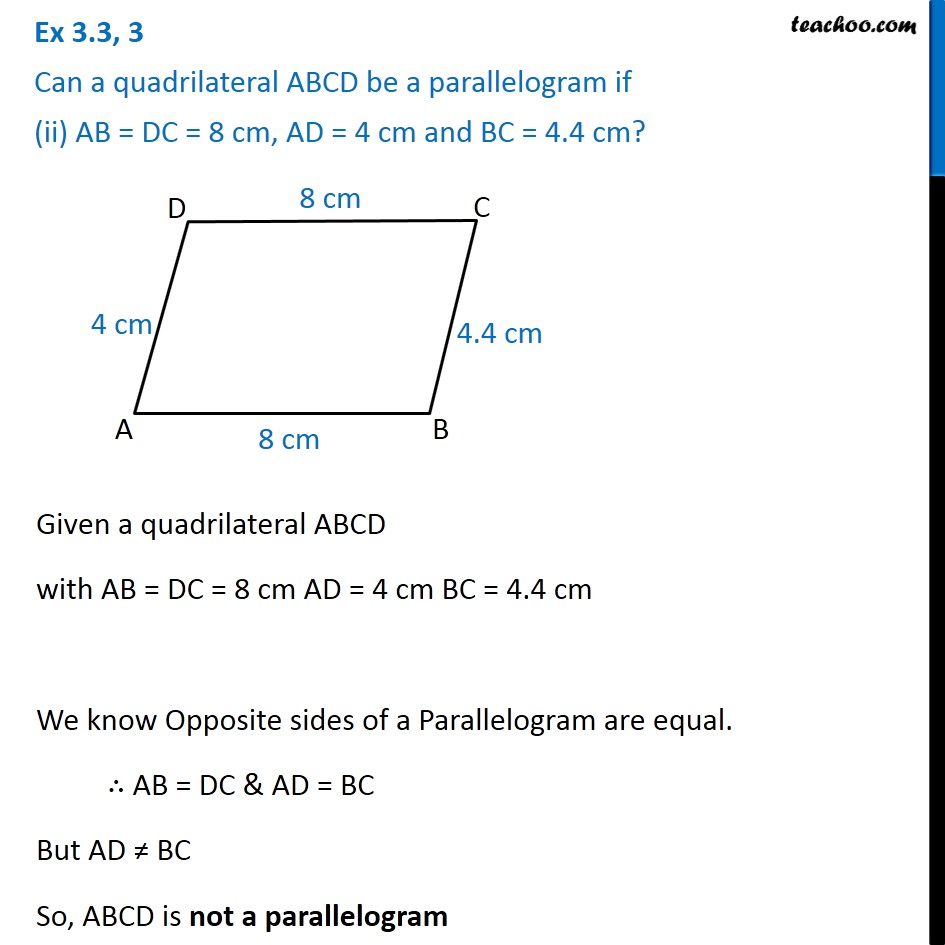Ex 3.3

Chapter 3 Class 8 Understanding Quadrilaterals
Serial order wiseLearn in your speed, with individual attention - Teachoo Maths 1-on-1 Class

### Transcript

Ex 3.3, 3 Can a quadrilateral ABCD be a parallelogram if (ii) AB = DC = 8 cm, AD = 4 cm and BC = 4.4 cm? Given a quadrilateral ABCD with AB = DC = 8 cm AD = 4 cm BC = 4.4 cm We know Opposite sides of a Parallelogram are equal. ∴ AB = DC & AD = BC But AD ≠ BC So, ABCD is not a parallelogram